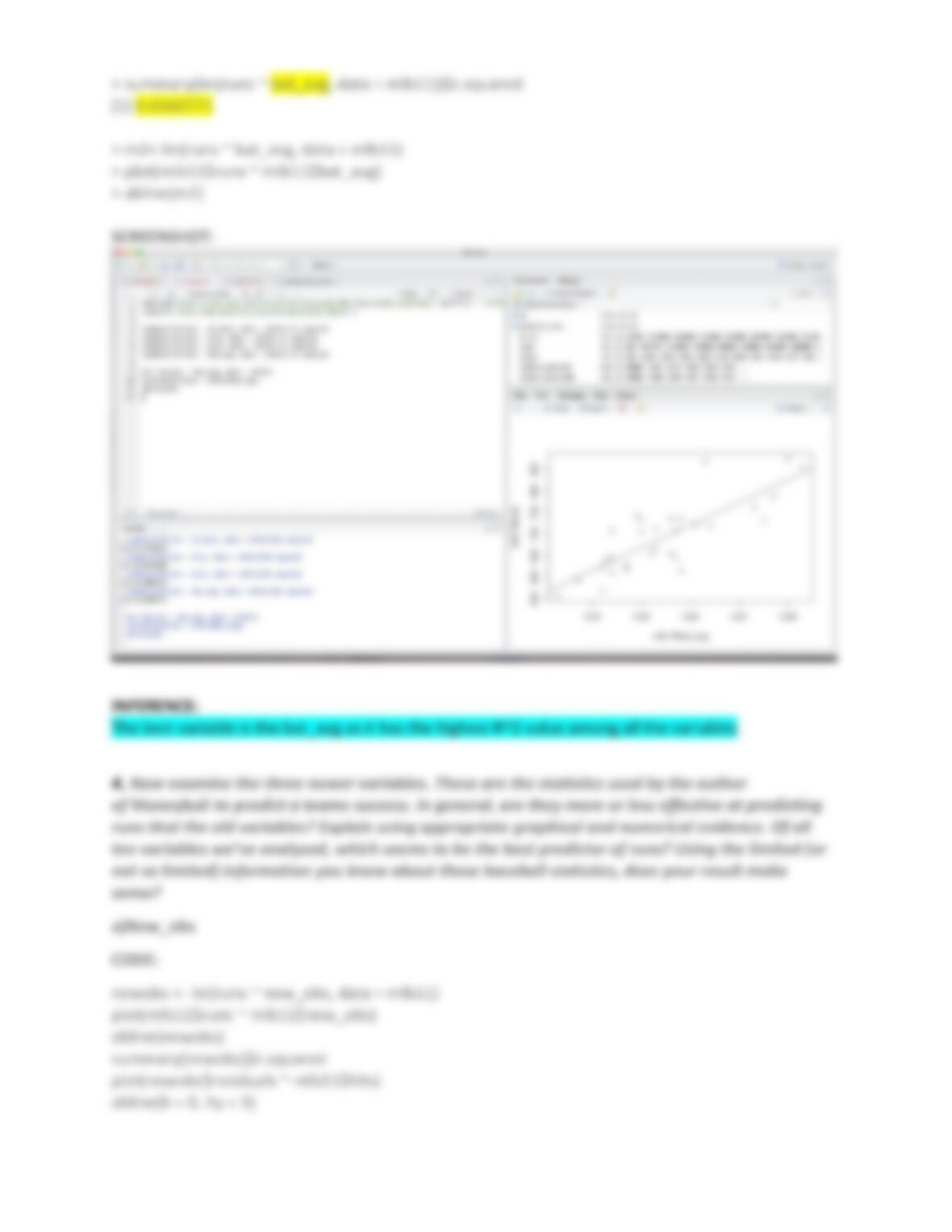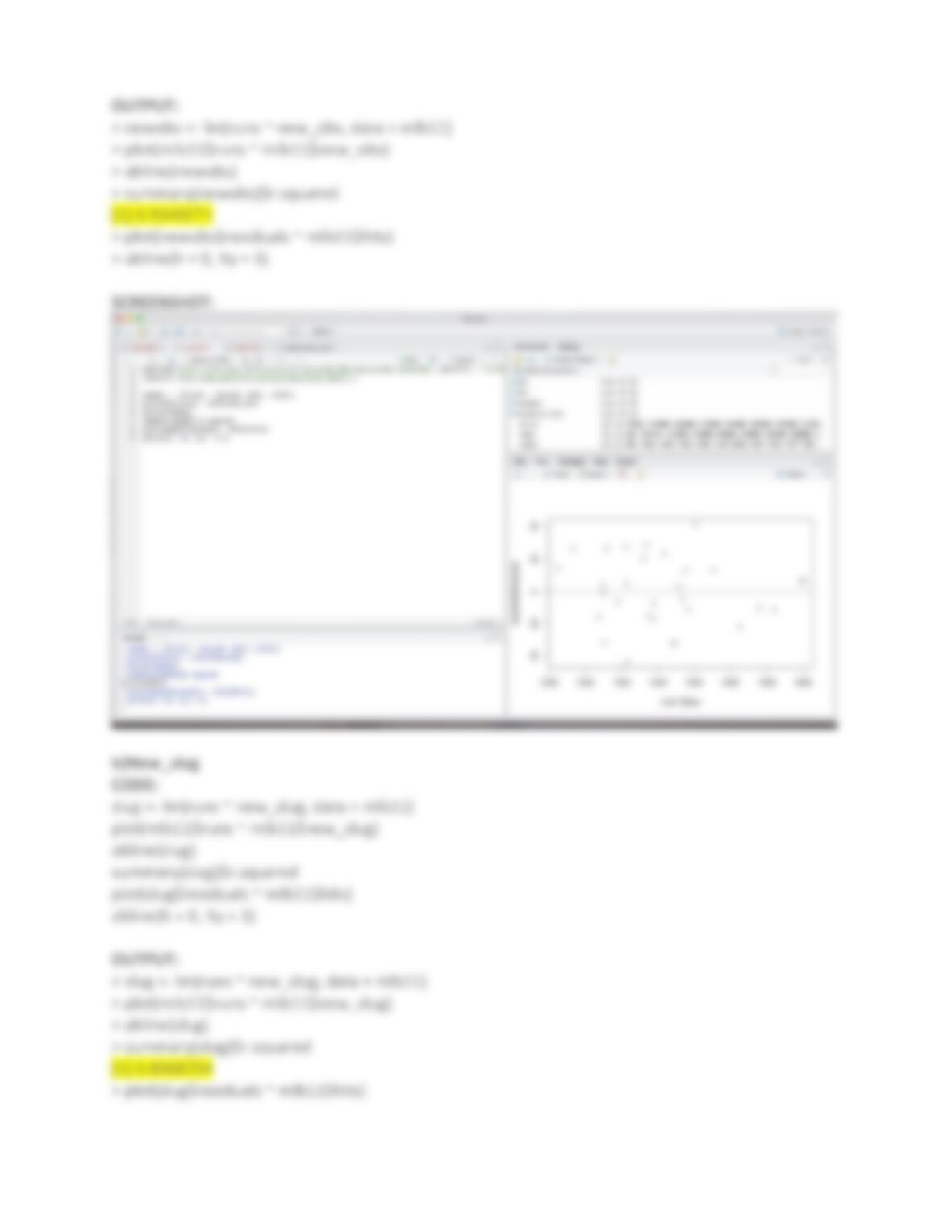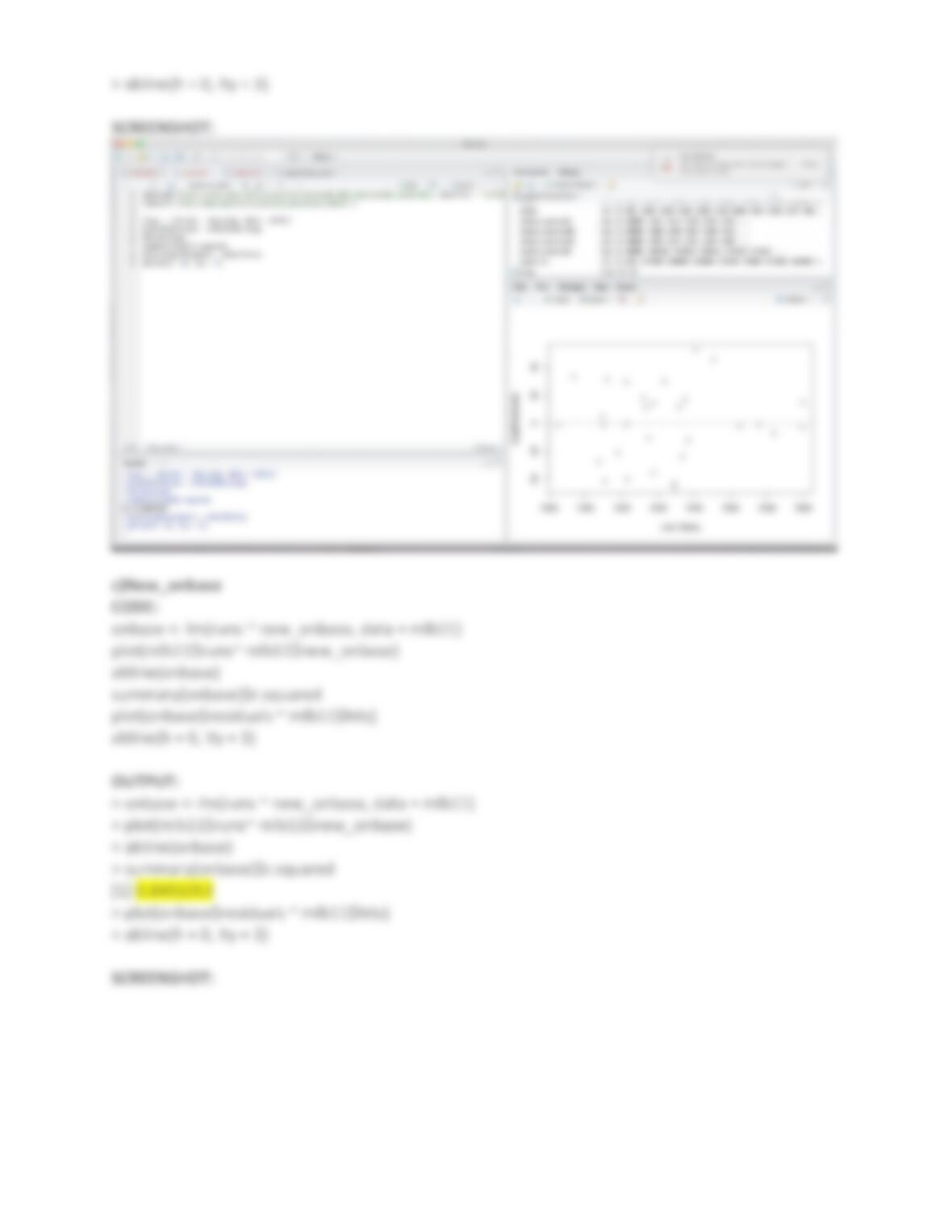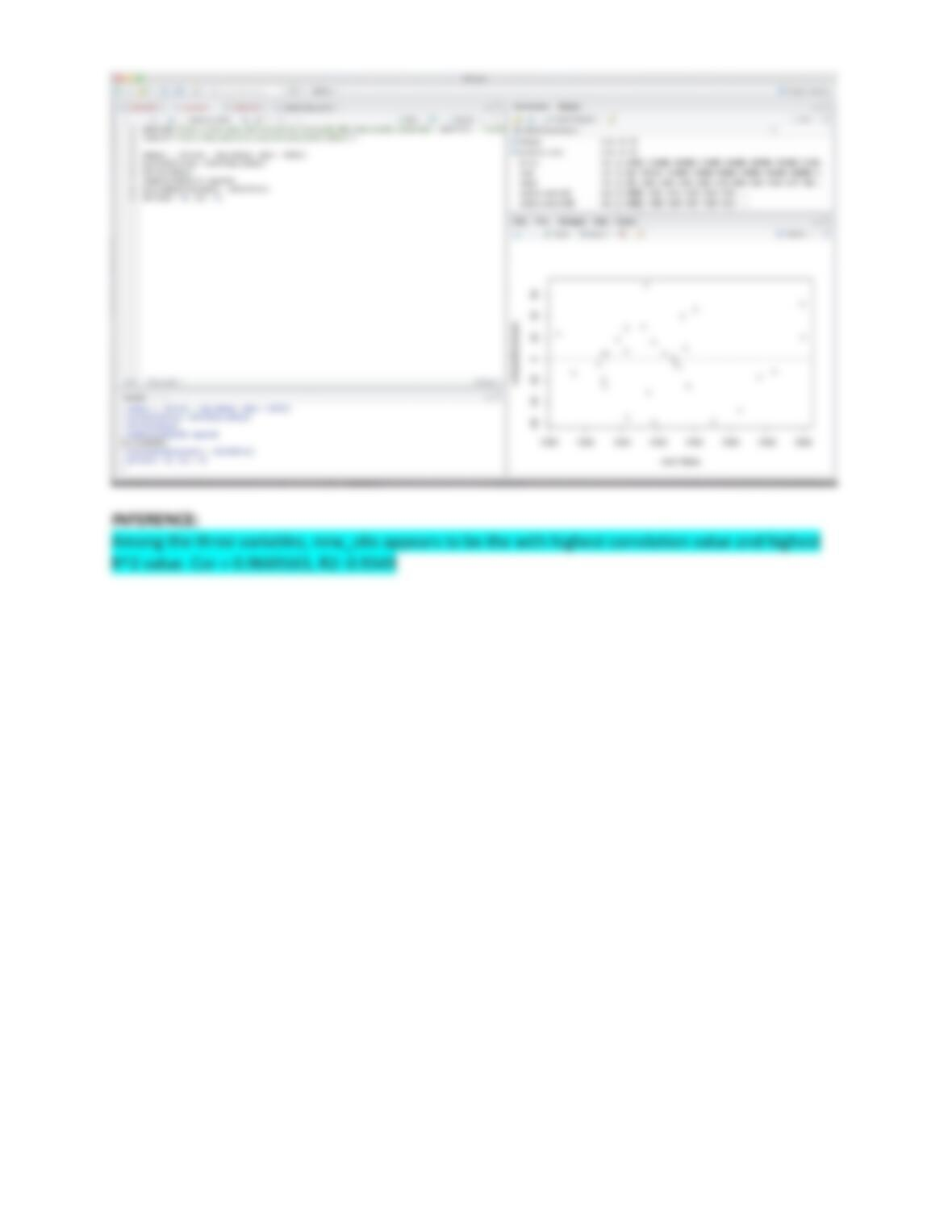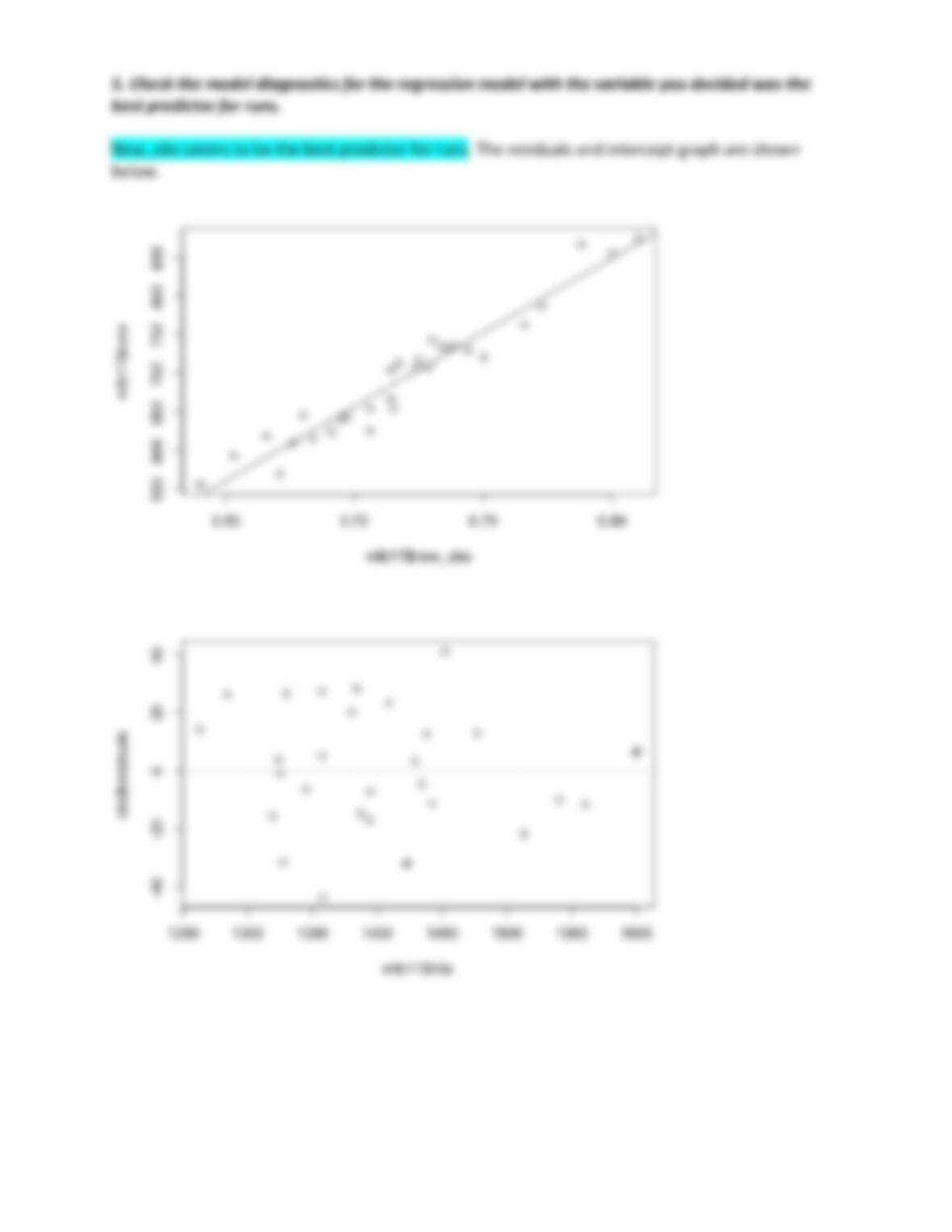Linear Regression

Type
Essay
Pages
8
Word Count
741
School
N/A
Course
N/A
Introduction to linear regression
1.Choose another traditional variable from mlb11 that you think might be a good predictor
of runs. Produce a scaerplot of the two variables and t a linear model. At a glance, does there
seem to be a linear relationship?
CODE:
predict_runs <- lm(runs ~ hits, data = mlb11)
predict_runs
plot(mlb11\$runs ~ mlb11\$hits)
abline(predict_runs)
plot(predict_runs\$residuals ~ mlb11\$hits)
abline(h = 0, lty = 3)
OUTPUT:
> predict_runs <- lm(runs ~ hits, data = mlb11)
> predict_runs
Call:
lm(formula = runs ~ hits, data = mlb11)
coefficient:
(Intercept) hits
-375.5600 0.7589
> plot(mlb11\$runs ~ mlb11\$hits)
> abline(predict_runs)
SCREENSHOT: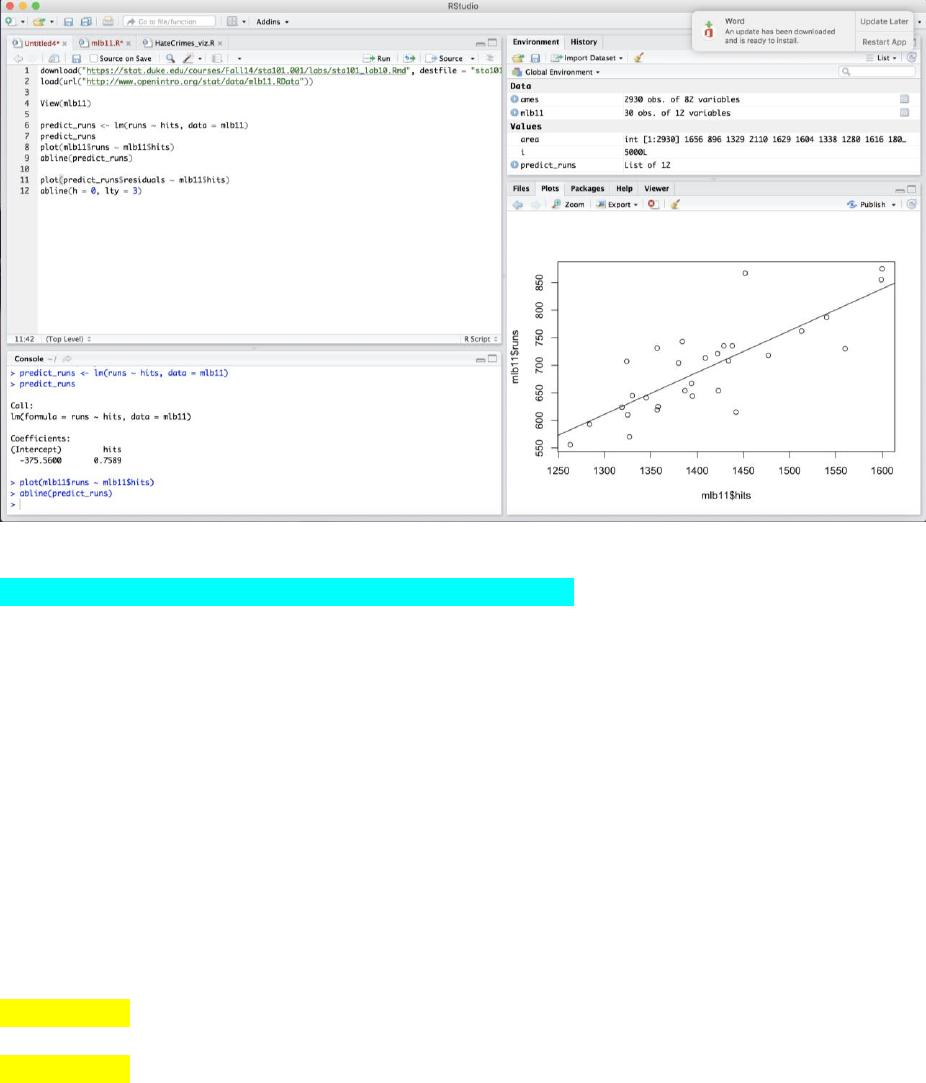INFERENCE:
Yes, there seems to be a linear rela,onship between the two.
2. How does this relationship compare to the relationship between runs and at_bats? Use the
R2 values from the two model summaries to compare. Does your variable seem to
predict runs beer than at_bats? How can you tell?
CODE:
m1 <- lm(runs ~ at_bats, data = mlb11)
summary(m1)\$r.squared
summary(predict_runs)\$r.squared
OUTPUT:
> m1 <- lm(runs ~ at_bats, data = mlb11)
> summary(m1)\$r.squared
 0.3728654
> summary(predict_runs)\$r.squared
 0.6419388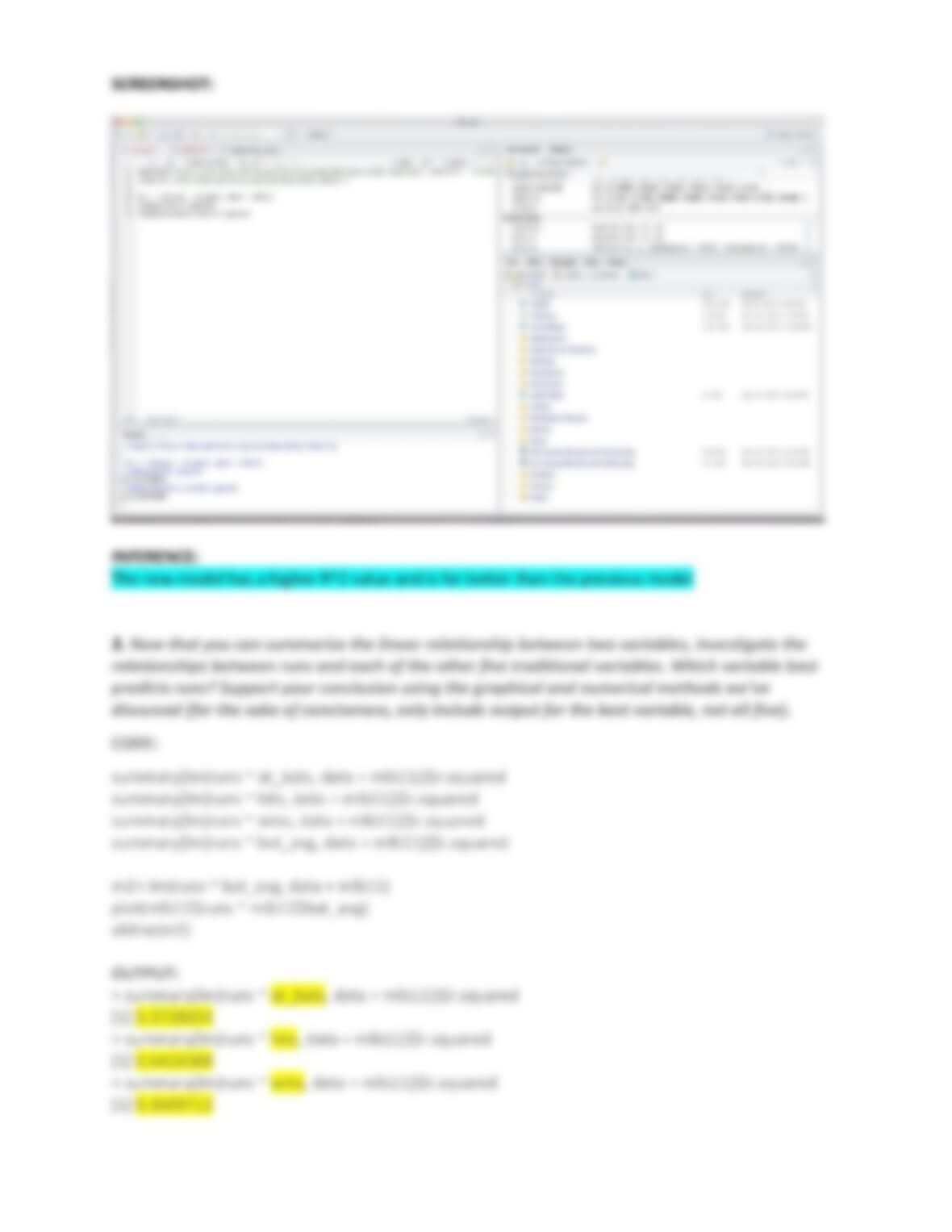SCREENSHOT:
INFERENCE:
The new model has a higher R^2 value and is far be8er than the previous model
3. Now that you can summarize the linear relationship between two variables, investigate the
relationships between runs and each of the other ve traditional variables. Which variable best
predicts runs? Support your conclusion using the graphical and numerical methods we’ve
discussed (for the sake of conciseness, only include output for the best variable, not all ve).
CODE:
summary(lm(runs ~ at_bats, data = mlb11))\$r.squared
summary(lm(runs ~ hits, data = mlb11))\$r.squared
summary(lm(runs ~ wins, data = mlb11))\$r.squared
summary(lm(runs ~ bat_avg, data = mlb11))\$r.squared
m2<-lm(runs ~ bat_avg, data = mlb11)
plot(mlb11\$runs ~ mlb11\$bat_avg)
abline(m2)
OUTPUT:
> summary(lm(runs ~ at_bats, data = mlb11))\$r.squared